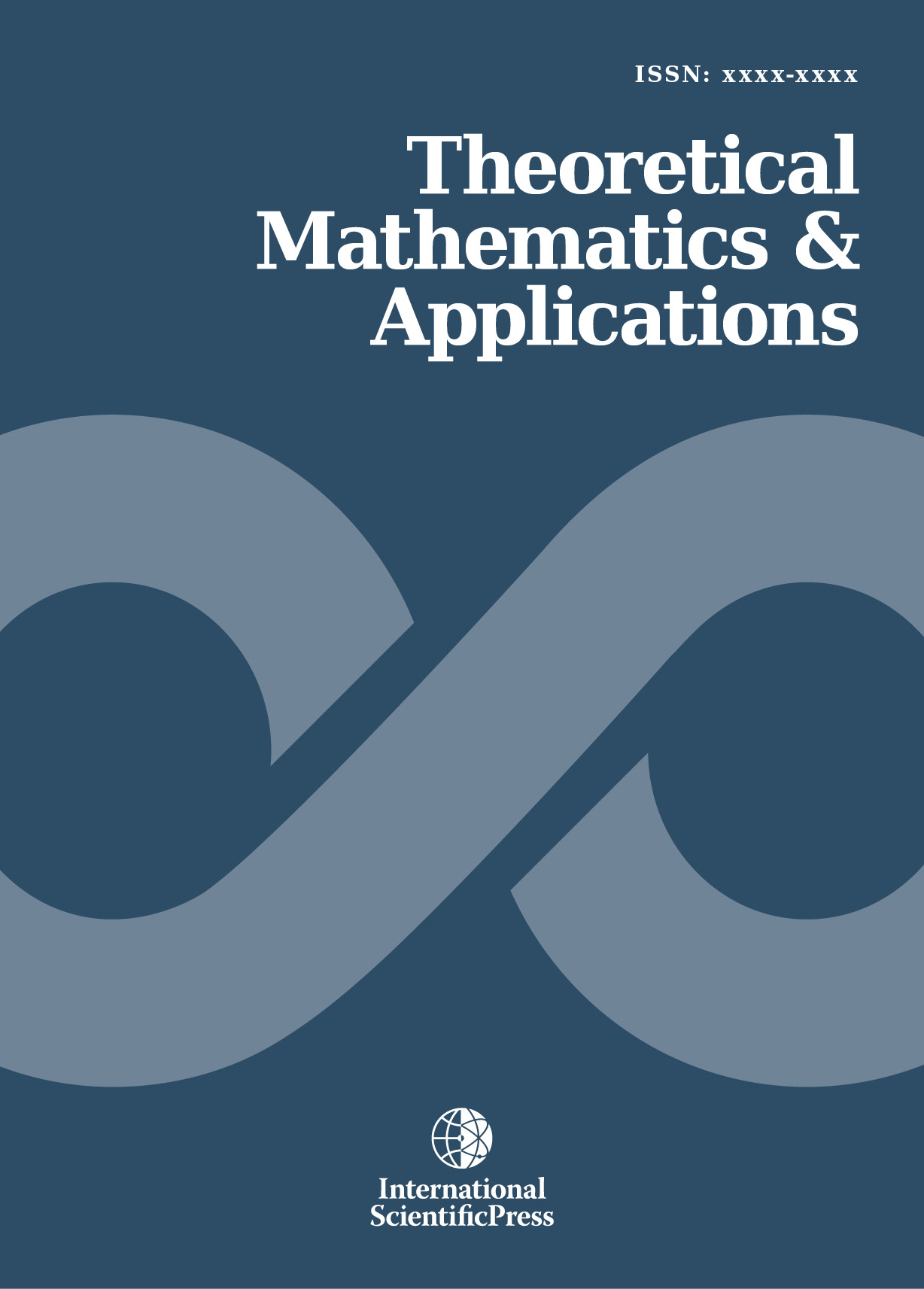# Theoretical Mathematics & Applications

#### Derivation of Extended Optimal Classification Rule for Multivariate Binary Variables

•[ Download ]
• Times downloaded: 677
• Abstract

The statistical classification of N individuals into G mutually exclusive groups when the actual group membership is unknown is common in the social and behavioral sciences. The result of such classification methods often has important consequences. Let π1 and π2 be two distinct r-variate Bernoulli populations. Given an object Φ with observation measurement vector (Xφ1,…Xφr), the optimal classification rule was developed to assign Φ to either π1 or π2. The performance of the optimal classification rule was compared with some existing procedures. The classification procedures are Full Multinomial, Predictive, Linear discriminant function. The expected cost of Misclassification was also derived. The four classification procedures for binary variables were discussed and evaluated at each of 118 configurations of the sampling experiments. The results obtained ranked the procedures as follows: Optimal, Linear discriminant, predictive and full multinomial rule. Further analysis revealed that increase in sample size and number of variables increase classification accuracy and lower the probability of Misclassification. Also apparent error rate obtained using resubstitution method is optimally biased and the bias decreases as the sample sizes increases.ISSN: 1792-9687 (Print)
1792-9709 (Online)